Back to Adaptive Vision Studio website

You are here: Start » Filter Reference » Region Features » RegionPerimeterLength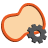# RegionPerimeterLength

Computes the length of the input region perimeter.

Name Type Description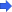inRegion Region Input region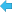outPerimeterLength Real

### Description

The operation computes the perimeter length of a region shape. Because regions are pixel-precise, the literal computation of the length of the region contour would lead to the overestimation of the diagonal edges. Therefore, the filter employs the following equation to approximate the result: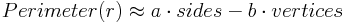Where

• sides denotes the number of pixel sides adjacent to the region background
• vertices is the number of turns to right during clockwise walk along the pixel sides of the region boundary
• a, b are constants defined as follows: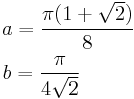To compute the actual contour of the region, one can use RegionContours filter.

### Examples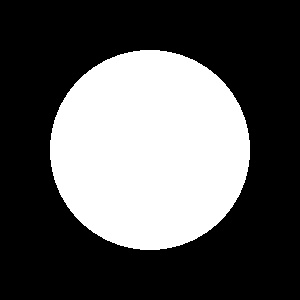RegionPerimeterLength run on the sample region (circular region of radius 100) computes outPerimeterLength = 627.382, while the actual perimeter of the perfect circle of radius 100 is 628.318 .

### Complexity Level

This filter is available on Basic Complexity Level.